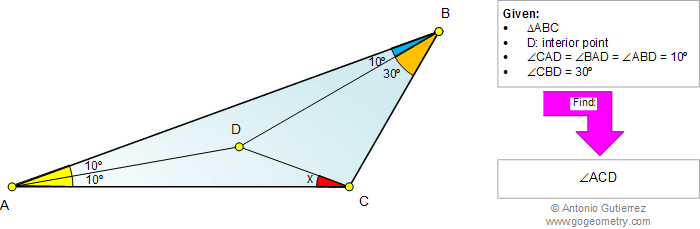# Geometry Problem 920: Triangle, Angles, 10, 20, 30 Degrees, Congruence. Level: High School, College, Mathematics Education

< PREVIOUS PROBLEM  |  NEXT PROBLEM >

 A point D is inside a triangle ABC (figure below) such that angle CAD = angle BAD = angle ABD = 10 degrees, angle CBD = 30 degrees. Find the measure of angle ACD. This entry contributed by Erina-NJ.Home | Search | Geometry | Problems | All Problems | Open Problems | Visual Index | 10 Problems | Problems Art Gallery |  Art | 911-920 | Triangles | Angles | Congruence | by Antonio Gutierrez